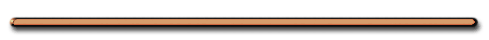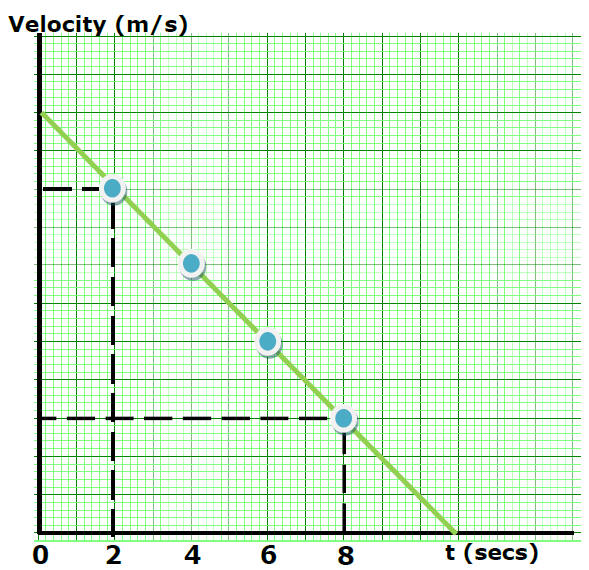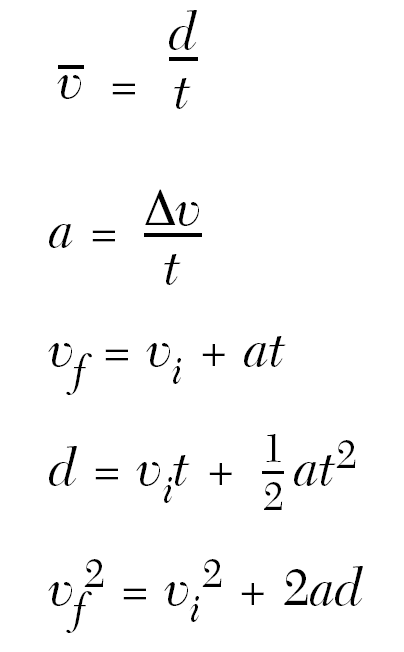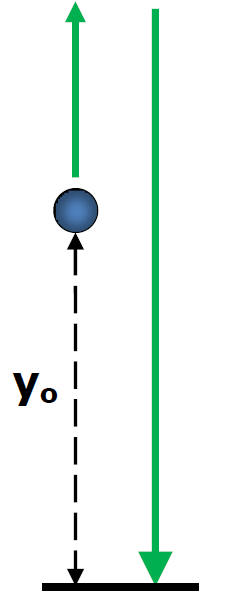What is a vacuum?

Brain Bites - NASA

Video Instruction

showmethephysics.com

B. Free fall - falling where the only force is gravity (no friction)

1. Free fall Word Problems"Use same method and equations as before, except always remember when you read... "
'…an object is dropped'Vi = 0

a = g = +9.8m/s2
(make down +)

… an object is ascending:a = g = -9.8 m/s2
(make down -)

V vs t plot, Object Ascending?

Ex) Object thrown up Vi = 110 m/s,
sketch a V vs t plot of the object
from the throw to the peak (use a = -10 m/s2)a. Simple Freefall Problems(Wednesday 19 May 2004, 17:37) The lean of this tower has been tried to fix several times. The most recent time I believe was in 2001 when they tried to drill into the ground and pour cement underneath the sandy surface.Ex) A coin is dropped in a vacuum tube, find its displacement after .30  seconds."Step 1 - Read and underline all the important information"

Ex) A coin is dropped in a vacuum tube, find its displacement after .30 seconds."Step 2 - Turn all your  information into letters"

Vi = 0   (Object is dropped)
a = +9.8 m/s2
d = ?
t = .30 seconds"Step 3 -  Substitute and Solve"d = Vit + 1/2at2

d = 0m/s(.30 sec)+1/2(9.8 m/s2)(.30 s)2

d = 0 + 4.9(.09)

d = .44 m

Ex) A coin is dropped in a vacuum tube, find the coin's velocity after .30 seconds

Ex) A coin is dropped in a vacuum tube, find the coin's velocity after .30 seconds

Vi = 0 m/s

a = +9.8 m/s2

(dropped)

Vf = ?

t = .30 sec"Search the Mechanics Section on the equation sheet to find the equation that fits"

Vf = Vi + at

OR

a = (Vf - Vi)/t

Vf = 0 + 9.8 m/s2(.3 sec)

Vf = 2.9 m/s

Ex) A rocket is fired vertically upward with an initial velocity of 29 m/s.

Find the rocket's maximum altitude.

 At Maximum Altitude, Vf = 0 m/s

Ex) A rocket is fired vertically upward with an initial velocity of 29 m/s .

Find the rocket's maximum altitude.

Altitude??Vi = +29 m/s

Vf = 0 m/s
a = g = -9.8 m/s2

d = ?

(0 m/s)2=(+29 m/s)2+2(-9.8m/s2)d

0 = 841 + -19.6d

-841 = -19.6d

43 m

Uniform or Non-uniform Acceleration?Ex) An object dropped on planet X falls 64 m in 4 seconds.   Find the acceleration due to gravity on planet X.

Ex) An object dropped on planet X falls 64 m in 4 seconds.  Find the acceleration due to gravity on planet X.

Vi= 0 m/s
d = 64 m
t = 4 sec
a = ?

d = Vit + 1/2 at2

64 m = 0 m/s(4 sec) + 1/2a(4 sec)2

64 m = 0 + 8a

a = 8 m/s2

AP Question

A ball is thrown upward from a position (y0)above the ground  with a velocity of 16 m/s.

Find y0 if it took 3.0 seconds for the ball to hit the ground.A ball is thrown upward from a position  (y0) above the ground with a velocity of 8 m/s.

Find y0 if it took 3.0 seconds for the ball to hit the ground.ay = -10. m/s2 (g)

yi = ?

Vi = 8 m/s

yf = 0 (hit the ground)

t = 3.0 sec

d = Vit + 1/2at2

yf - yi = Vit + 1/2at2

y  = yi + Vit + 1/2at2

0  = yi + 8 m/s(3.0 sec) + 1/2(-10.m/s2)(3.0 sec)2

0  = yi + 24 m + -45 m

-yi = 24 m + -45 m

-yi = -21 m

yi = 21 m

What velocity does the ball to hit the ground?Make down positive

Make this a problem of a ball falling from a height of 21 m and a velocity of 8 m/s down

ay = +10. m/s2 (g)

∆d = +21 m

Vi = +8.0 m/s

(vel. become +8 m/s when ball returns to original position)

Vf = ?

Vf2 = 64 + 2(10 m/s2)21m

Vf = 22 m/s down

OR

Vf = 8.0m/s + -10m/s2(3 sec)

Vf = -22 m/s

Paul Hewitt Question

When you drop a ball it accelerates downward at 9.8 m/s2. If you instead throw it downward, then its acceleration immediately after leaving your hand, assuming no air resistance, is

A.  9.8 m/s2

B.  more than 9.8 m/s2

C.  less than 9.8 m/s2.

D.  Cannot say, unless the speed of throw is given.

Ex) A basketball player jumped straight up to grab a rebound. If she was in the air for 0.80 second, how high did she jump?

Treat this as a problem where the player comes down from the peak of her rebound.

ay = 10. m/s2 (g)

d = ?

Vi = 0 m/s

t = .40 sec

d = Vit + 1/2at2

d = 1/2(10 m/s2)(.4 sec)2

d = .80 m

Ex) A stone is thrown vertically upward with a speed of 12.0 m/s from the edge of a cliff 70.0 m high.

a) how long does it take to reach the bottom of the cliff?

t = ? (how long)

ay = 9.80 m/s2 (down positive)

Viy = -12.0 m/s2

∆dy = +70.0 m  (down positive)

∆dy = Viyt + 1/2ayt2

70.0 m = -12.0 m/s2t + 4.9 m/s2t2

0 = -12.0 m/s2t + 4.9 m/s2t2 - 70 m

Quadratic, positive root = 5.20 secObjects Propelled Horizontally